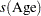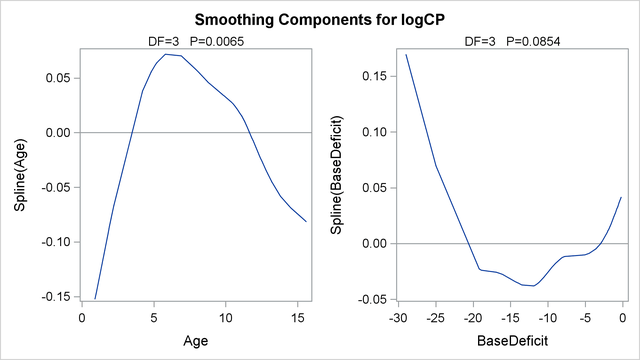Getting Started: GAM Procedure

The following example illustrates the use of the GAM procedure to explore in a nonparametric way how two factors affect a response. The data come from a study (Sockett et al. 1987) of the factors affecting patterns of insulin-dependent diabetes mellitus in children. The objective is to investigate the dependence of the level of serum C-peptide on various other factors in order to understand the patterns of residual insulin secretion. The response measurement is the logarithm of C-peptide concentration (pmol/ml) at diagnosis, and the predictor measurements are age and base deficit (a measure of acidity).

```title 'Patterns of Diabetes';
data diabetes;
input Age BaseDeficit CPeptide @@;
logCP = log(CPeptide);
datalines;
5.2    -8.1  4.8   8.8  -16.1  4.1  10.5   -0.9  5.2
10.6   -7.8  5.5  10.4  -29.0  5.0   1.8  -19.2  3.4
12.7  -18.9  3.4  15.6  -10.6  4.9   5.8   -2.8  5.6
1.9   -25.0  3.7   2.2   -3.1  3.9   4.8   -7.8  4.5
7.9   -13.9  4.8   5.2   -4.5  4.9   0.9  -11.6  3.0
11.8   -2.1  4.6   7.9   -2.0  4.8  11.5   -9.0  5.5
10.6  -11.2  4.5   8.5   -0.2  5.3  11.1   -6.1  4.7
12.8   -1.0  6.6  11.3   -3.6  5.1   1.0   -8.2  3.9
14.5   -0.5  5.7  11.9   -2.0  5.1   8.1   -1.6  5.2
13.8  -11.9  3.7  15.5   -0.7  4.9   9.8   -1.2  4.8
11.0  -14.3  4.4  12.4   -0.8  5.2  11.1  -16.8  5.1
5.1    -5.1  4.6   4.8   -9.5  3.9   4.2  -17.0  5.1
6.9    -3.3  5.1  13.2   -0.7  6.0   9.9   -3.3  4.9
12.5  -13.6  4.1  13.2   -1.9  4.6   8.9  -10.0  4.9
10.8  -13.5  5.1
;
```

The following statements perform the desired analysis. The PROC GAM statement invokes the procedure and specifies the diabetes data set as input. The MODEL statement specifies logCP as the response variable and requests that univariate smoothing splines with the default ofdegrees of freedom be used to model the effect of Age and BaseDeficit.

```ods graphics on;
proc gam data=diabetes;
model logCP = spline(Age) spline(BaseDeficit);
run;
```

The results are shown in Figure 38.1 and Figure 38.2.

Figure 38.1 Summary Statistics
 Patterns of Diabetes

The GAM Procedure
Dependent Variable: logCP
Smoothing Model Component(s): spline(Age) spline(BaseDeficit)

Summary of Input Data Set
Number of Observations 43
Number of Missing Observations 0
Distribution Gaussian

Iteration Summary and Fit Statistics
Final Number of Backfitting Iterations 5
Final Backfitting Criterion 5.542745E-10
The Deviance of the Final Estimate 0.4180791724

Figure 38.1 shows two tables. The first table summarizes the input data set and the distributional family used for the model; the second table summarizes the convergence criterion for backfitting.

Figure 38.2 Analysis of Model
Regression Model Analysis
Parameter Estimates
Parameter Parameter
Estimate
Standard
Error
t Value Pr > |t|
Intercept 1.48141 0.05120 28.93 <.0001
Linear(Age) 0.01437 0.00437 3.28 0.0024
Linear(BaseDeficit) 0.00807 0.00247 3.27 0.0025

Smoothing Model Analysis
Fit Summary for Smoothing Components
Component Smoothing
Parameter
DF GCV Num
Unique
Obs
Spline(Age) 0.995582 3.000000 0.011675 37
Spline(BaseDeficit) 0.995299 3.000000 0.012437 39

Smoothing Model Analysis
Analysis of Deviance
Source DF Sum of Squares Chi-Square Pr > ChiSq
Spline(Age) 3.00000 0.150761 12.2605 0.0065
Spline(BaseDeficit) 3.00000 0.081273 6.6095 0.0854

Figure 38.2 displays summary statistics for the model. It consists of three tables. The first is the "Parameter Estimates" table for the parametric part of the model. It indicates that the linear trends for both Age and BaseDeficit are highly significant. The second table is the summary of smoothing components of the nonparametric part of the model. By default, each smoothing component has approximately 4 degrees of freedom (DF). For univariate spline components, one DF is taken up by the (parametric) linear part of the model, so the remaining approximate DF is 3, and the main point of this table is to present the smoothing parameter values that yield this DF for each component. Finally, the third table is the "Analysis of Deviance" table for the nonparametric component of the model.

Graphical displays are produced when ODS Graphics is enabled. By default, the graphics features of PROC GAM produce plots of the partial predictions of each variable. In these plots, the partial prediction for a predictor such as Age is its nonparametric contribution to the model,. For general information about ODS Graphics, see Chapter 21, Statistical Graphics Using ODS. For specific information about the graphics available in the GAM procedure, see the section ODS Graphics.

Plots for both predictors (Figure 38.3) show a strong quadratic pattern, with a possible indication of higher-order behavior. Further investigation is required to determine whether these patterns are real or not.

Figure 38.3 Partial Predictions for Each Predictor